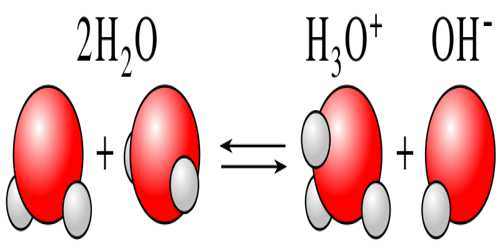# Self-Ionization (or autoionization) of Water

Self-Ionization (or autoionization) of Water

Water is known to be a non-electrolyte (non-conductor of electricity), although precise measurements indicate that water is a weak conductor of electricity. The conductivity of water arises due to a phenomenon is known as self-ionization. In water two molecules of water may react to give ions as shown below:

H2O (l) + H2O (l) ↔ H3O+ (aq) + OH (aq)

The equilibrium constant for self-ionization of eater is given by,

K = {[H3O+] [OH]} / [H2O]2 …. …. (1)

Since the concentration of the ions formed by self-ionization is very small, the concentration of H2O remains practically constant and equation (1) can be rewritten as,

[H2O]2 x K = [H3O+] x [OH] …. …. (2)

The quantities on the left-hand side of equation (2) are constants and maybe, replaced by a new constant term KW, known as the ionic product of water.

KW = [H3O+] x [OH] …. …. (3)KW is a very important quantity of water. In all ionic equilibrium in water, KW is constant as long as the temperature is constant. In pure water

[H3O+] (or simply [H+]) = [OH]

so that, KW = [H+]2 = [OH]2 … …. …. (4)

The value of KW has been carefully determined at different temperatures by various methods. The value of KW at 298 K is about 1.0 x10-14 mol2 L-6. Whether the solution is acidic or alkaline the value of [H+] x [OH] will always be 1.0 x 10-14 at 298 K. The ionic product KW of water may be calculated from conductance data as follows:

The specific conductance of pure water at 298 K is 5.50 x 10-8 ohm-1 cm-1. The ion conductances λ0H+ and λ0OH- at 298 K and at infinite dilutions are 349.8 cm2 ohm-1mol-1 and 198.0 cm2 ohm-1mol-1 respectively. So the molar conductance is-

Λ0 = λ0H+ + λ0OH-

= [349.8 cm2 ohm-1mol-1] + [198.0 cm2 ohm-1mol-1]

Using equation,

C = 1000k / Λ0 = (1000 x 5.5 x 10-8) / 547.8 = 1.00 x 10-7

where c is the concentration of ionized water, As each molecule of H2O gives one H+ ion and one OH ion we can write;

c = [H+] = [OH]

That is to say that in pure water the concentrations of H+ and OH are equal.

Hence,

KW = [H+]2 = (1.00 x 10-7)2

= 1.0 x 10-14

The ionic product of water at various temperatures is given in Table.

Table: Values of KW at different temperatures